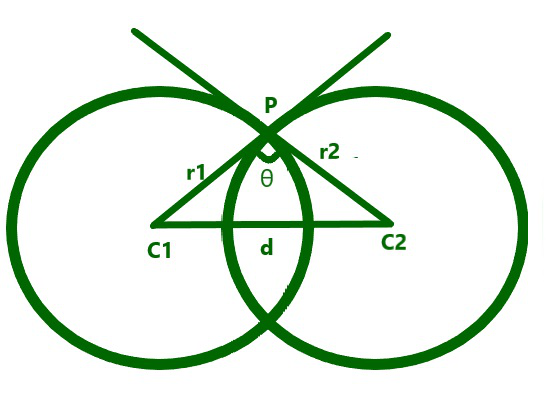# Angle of intersection of two circles having their centers D distance apart

• Last Updated : 16 Jul, 2021

Given two positive integers R1 and R2 representing the radius of two intersecting circles having a distance D between their centers, the task is to find the cosine of the angle of intersection between the two circles.

Examples:

Attention reader! Don’t stop learning now. Get hold of all the important DSA concepts with the DSA Self Paced Course at a student-friendly price and become industry ready.  To complete your preparation from learning a language to DS Algo and many more,  please refer Complete Interview Preparation Course.

In case you wish to attend live classes with experts, please refer DSA Live Classes for Working Professionals and Competitive Programming Live for Students.

Input: R1 = 3, R2 = 4, D = 5
Output: 0

Input: R1 = 7, R2 = 3, D = 6
Output: 0.52381

Approach: The given problem can be solved by using the Geometric Algorithm as illustrated below:From the above image and using the Pythagoras Theorem, the cosine of the angle of intersection of the two circles can be found using the formula:Below is the implementation of the above approach:

## C++

 // C++ program for the above approach#include using namespace std; // Function to find the cosine of the// angle of the intersection of two// circles with radius R1 and R2float angle(float R1, float R2, float D){    float ans = (R1 * R1 + R2 * R2 - D * D)                / (2 * R1 * R2);     // Return the cosine of the angle    return ans;} // Driver Codeint main(){    float R1 = 3, R2 = 4;    float D = 5;    cout << angle(R1, R2, D);     return 0;}

## Java

 // Java program for the above approachimport java.io.*; class GFG{     // Function to find the cosine of the// angle of the intersection of two// circles with radius R1 and R2static float angle(float R1, float R2, float D){    float ans = (R1 * R1 + R2 * R2 - D * D) /               (2 * R1 * R2);                    // Return the cosine of the angle    return ans;} // Driver Codepublic static void main (String[] args){    float R1 = 3, R2 = 4;    float D = 5;         System.out.println(angle(R1, R2, D));}} // This code is contributed by Ankita saini

## Python3

 # Python3 program for the above approach # Function to find the cosine of the# angle of the intersection of two# circles with radius R1 and R2def angle(R1, R2, D):         ans = ((R1 * R1 + R2 * R2 - D * D) /            (2 * R1 * R2))     # Return the cosine of the angle    return ans # Driver Codeif __name__ == '__main__':         R1 = 3    R2 = 4    D = 5         print(angle(R1, R2, D))     # This code is contributed by ipg2016107

## C#

 // C# program for the above approachusing System; class GFG{     // Function to find the cosine of the// angle of the intersection of two// circles with radius R1 and R2static float angle(float R1, float R2, float D){    float ans = (R1 * R1 + R2 * R2 - D * D) /               (2 * R1 * R2);                    // Return the cosine of the angle    return ans;} // Driver Codepublic static void Main(string[] args){    float R1 = 3, R2 = 4;    float D = 5;         Console.Write(angle(R1, R2, D));}} // This code is contributed by rutvik_56.

## Javascript

 
Output:
0

Time Complexity: O(1)
Auxiliary Space: O(1)

My Personal Notes arrow_drop_up